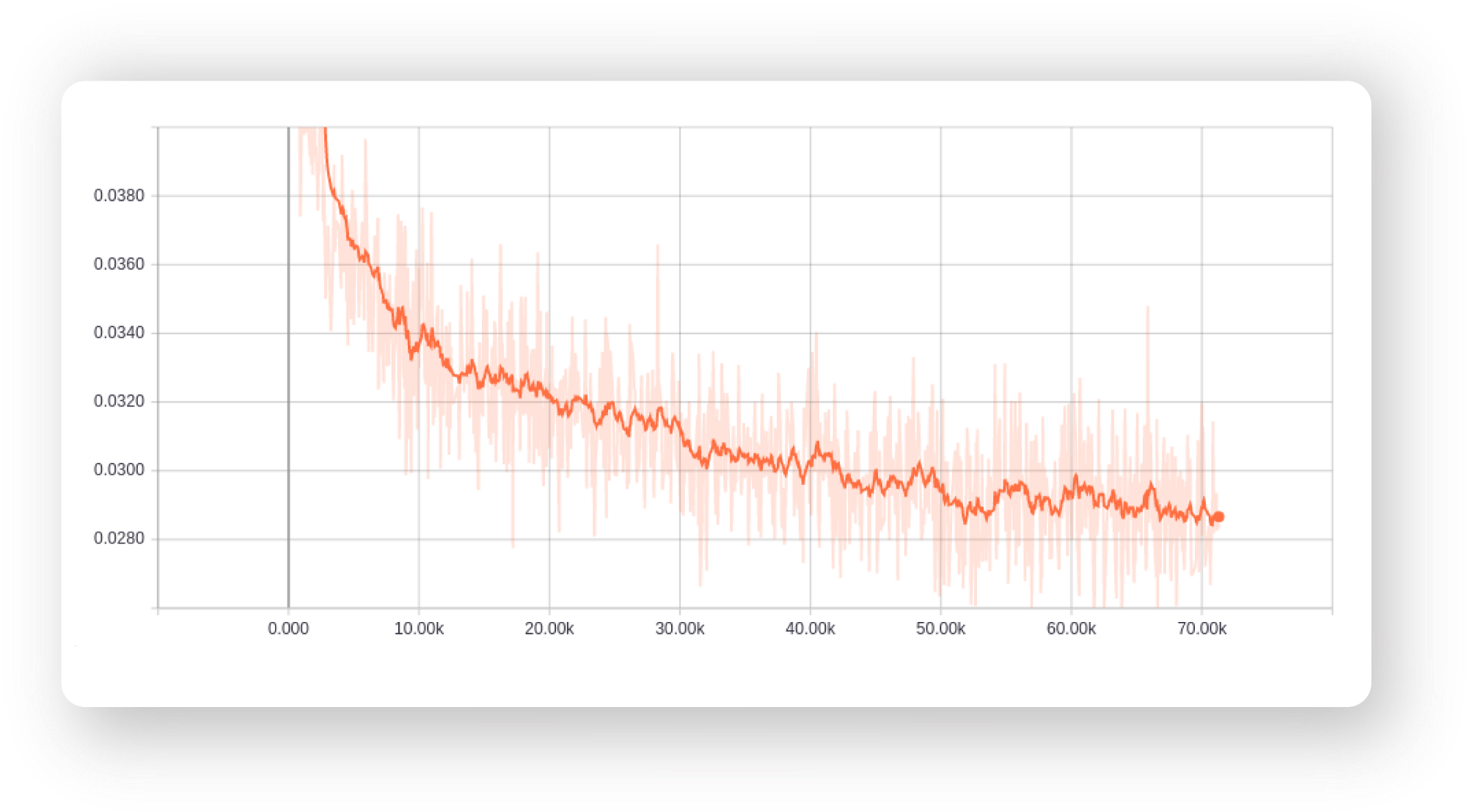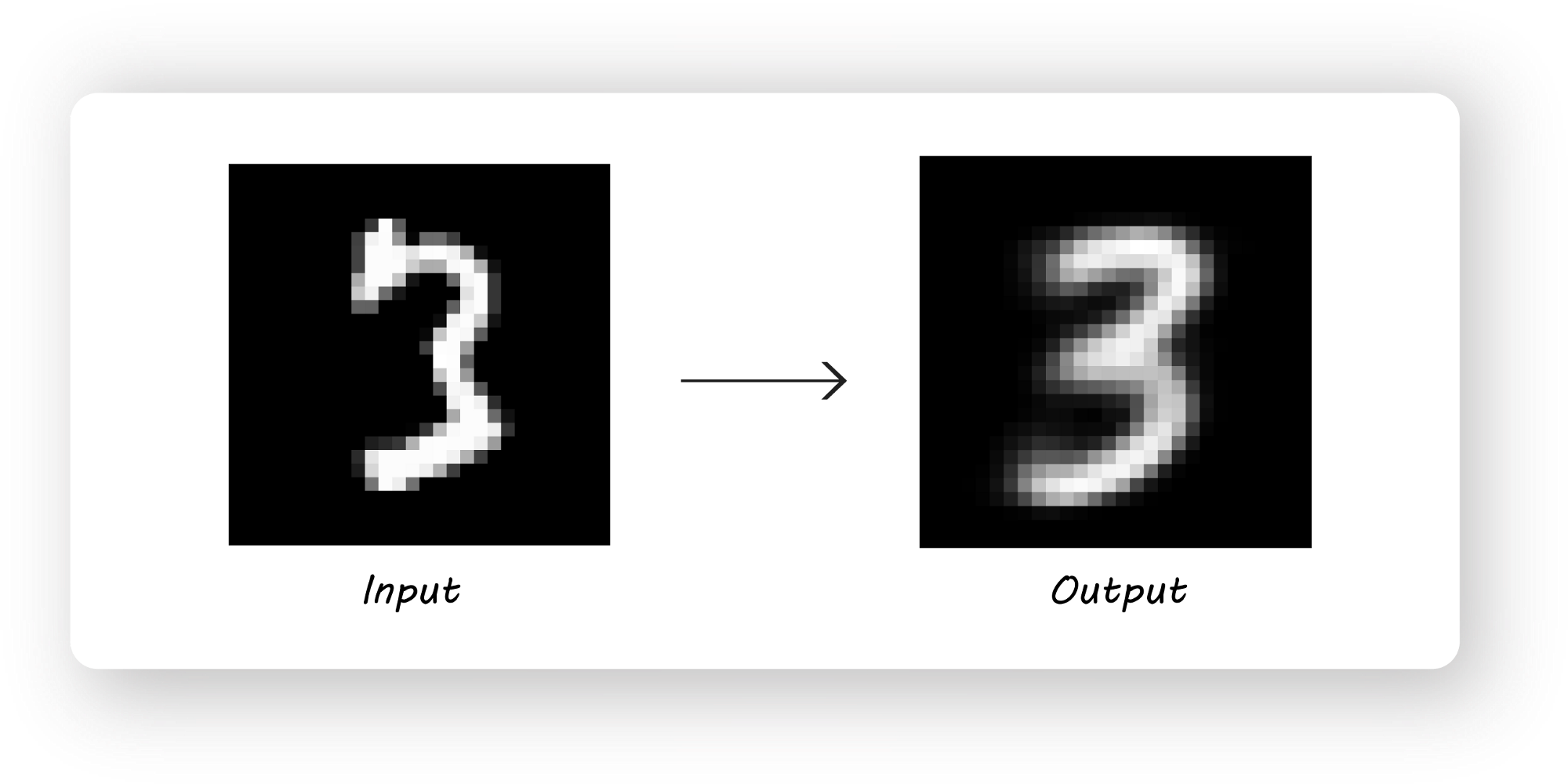# 对抗自编码器指南之一：自编码器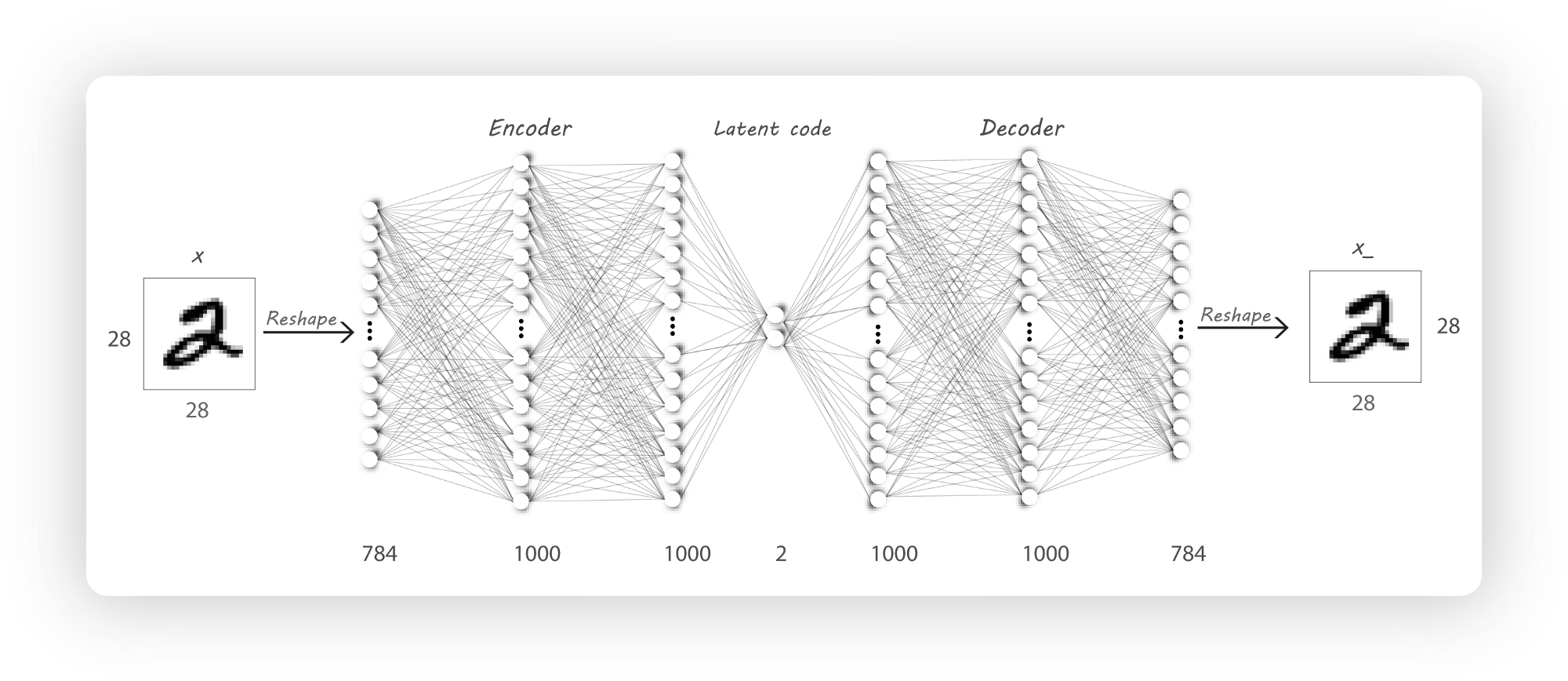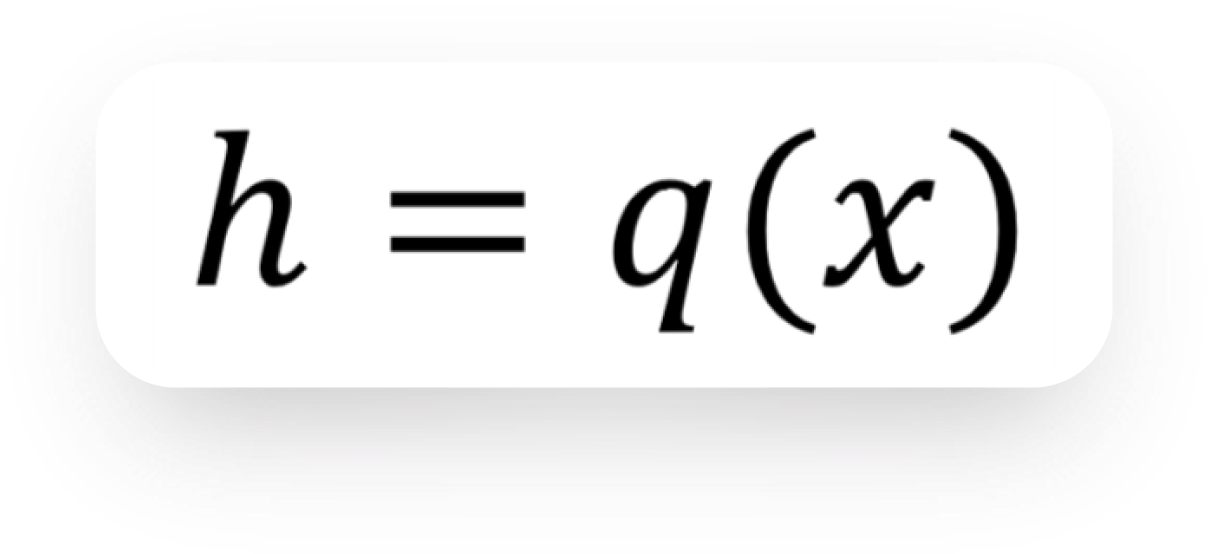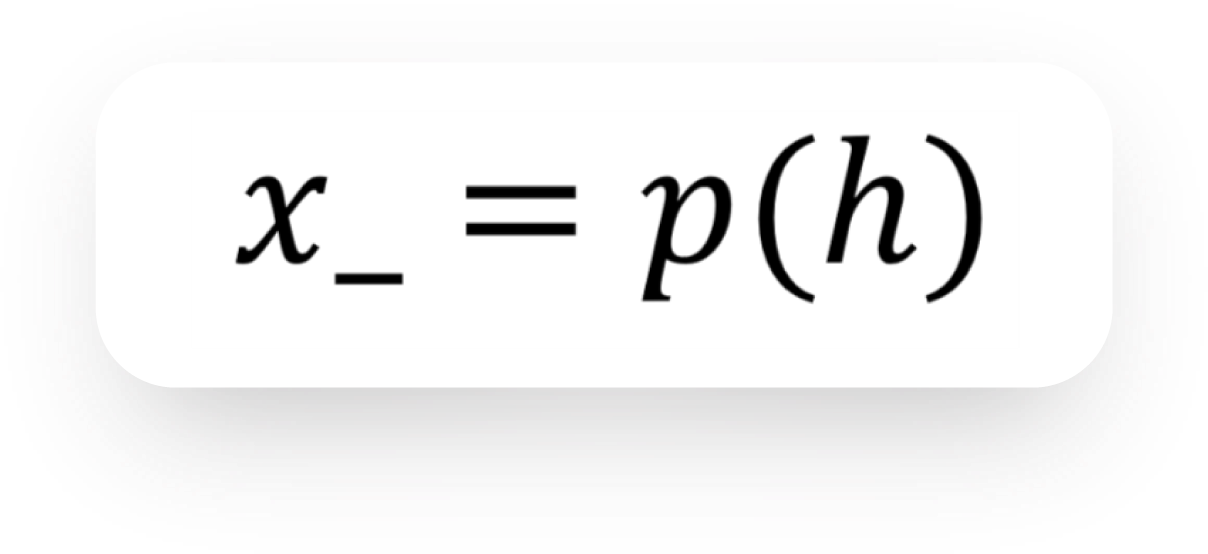• 图像去噪：输入有噪声的图像，输出清晰的无噪声图像。下图是手写数字去噪自编码器的例子：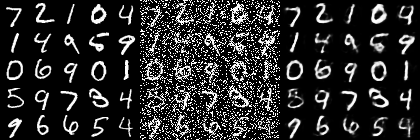• 语义散列技术通过降维使信息检索更快（我发现这非常有趣！）。
• 以对抗方式训练出的自编码器可以用作生成式（generative）模型，这一点我们将在后面进一步深入。

• 第1部分：自编码器

• 第2部分：探索对抗自编码器的隐空间

• 第3部分：分离样式与内容

• 第4部分：用1000个标签分类MNIST数据。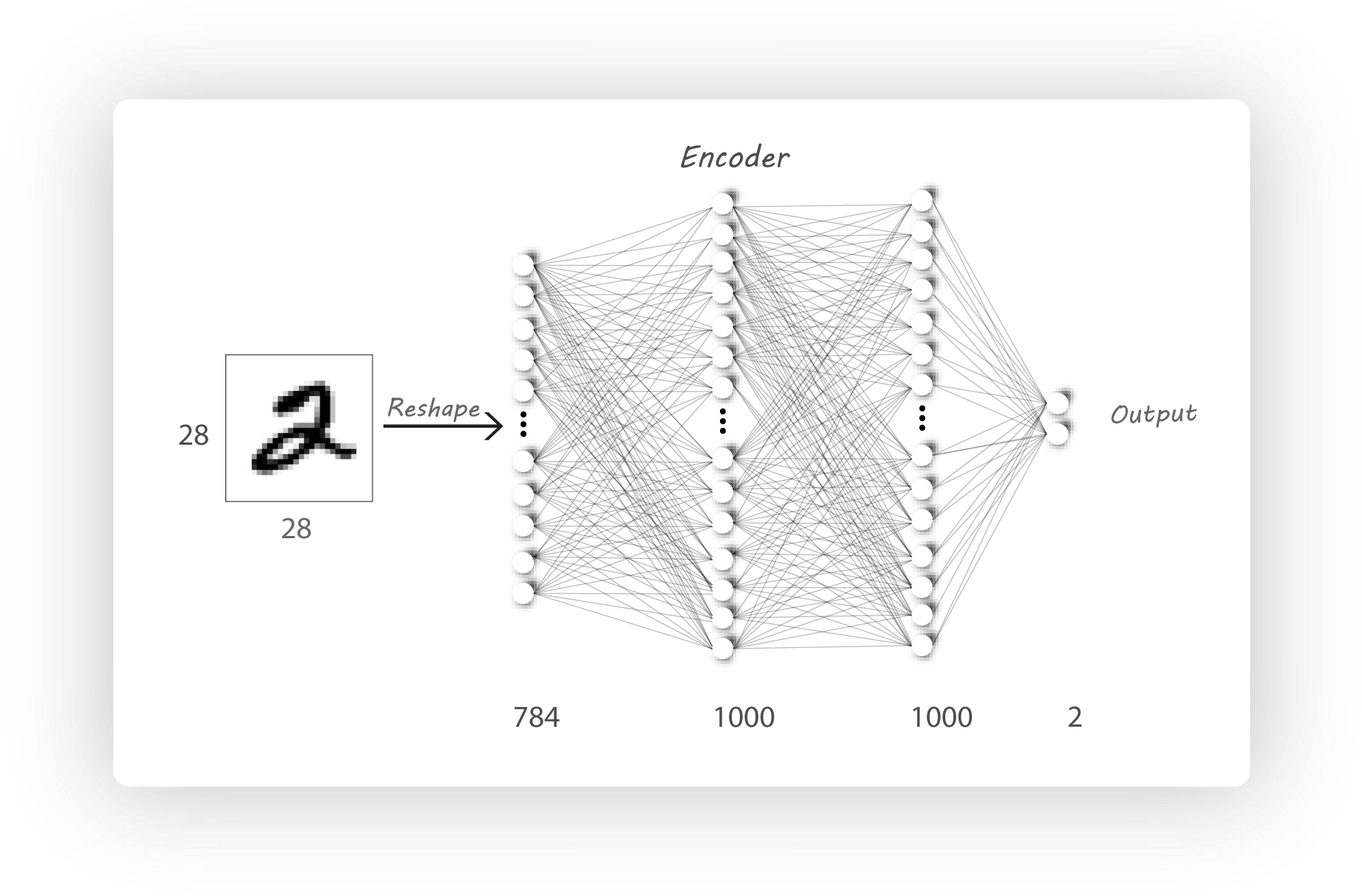def dense(x, n1, n2, name):
"""
Used to create a dense layer.
:param x: input tensor to the dense layer
:param n1: no. of input neurons
:param n2: no. of output neurons
:param name: name of the entire dense layer.i.e, variable scope name.
:return: tensor with shape [batch_size, n2]
"""
with tf.variable_scope(name, reuse=None):
weights = tf.get_variable("weights", shape=[n1, n2],
initializer=tf.random_normal_initializer(mean=0., stddev=0.01))
bias = tf.get_variable("bias", shape=[n2], initializer=tf.constant_initializer(0.0))
out = tf.add(tf.matmul(x, weights), bias, name='matmul')
return out

def encoder(x, reuse=False):
"""
Encode part of the autoencoder
:param x: input to the autoencoder
:param reuse: True -> Reuse the encoder variables, False -> Create or search of variables before creating
:return: tensor which is the hidden latent variable of the autoencoder.
"""
if reuse:
tf.get_variable_scope().reuse_variables()
with tf.name_scope('Encoder'):
e_dense_1 = tf.nn.relu(dense(x, input_dim, n_l1, 'e_dense_1'))
e_dense_2 = tf.nn.relu(dense(e_dense_1, n_l1, n_l2, 'e_dense_2'))
latent_variable = dense(e_dense_2, n_l2, z_dim, 'e_latent_variable')
return latent_variable
• reuse标志用于重用训练好的编码器。
• input_dim = 784, n_l1 = 1000, n_l2 = 1000, z_dim = 2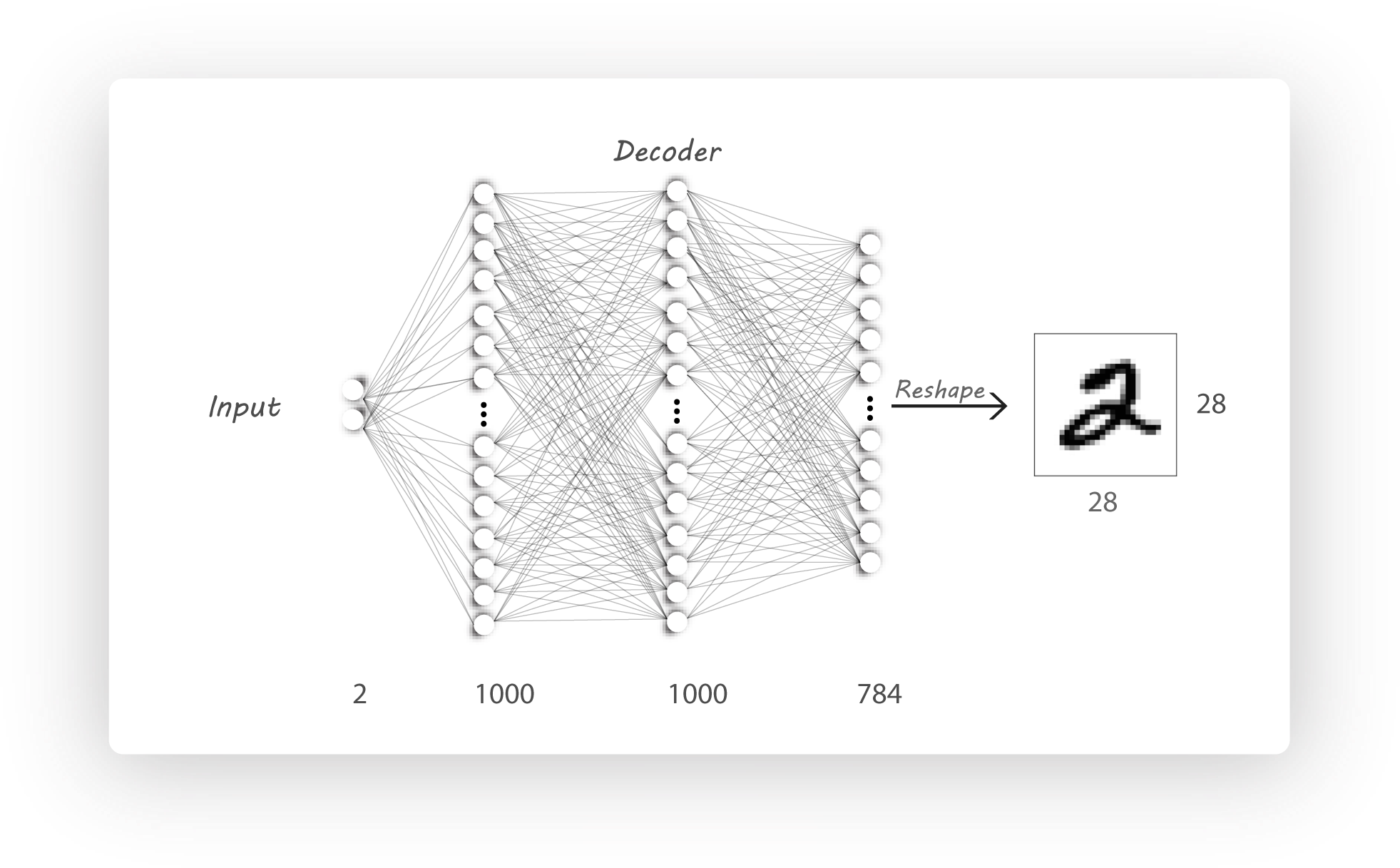• z_dim = 2, n_l2 = 1000, n_l1 = 1000, input_dim = 784，与编码器相同。

encoder_output = encoder(x_input)
decoder_output = decoder(encoder_output)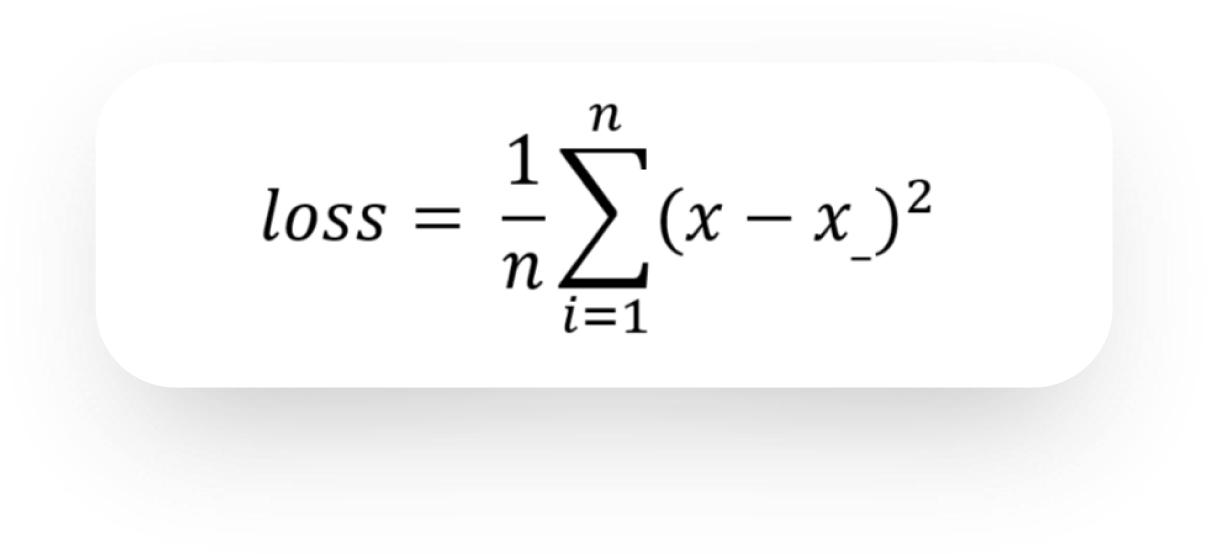loss = tf.reduce_mean(tf.square(x_target - decoder_output))

optimizer = tf.train.AdamOptimizer(learning_rate=learning_rate, beta1=beta1).minimize(loss)

step = 0
with tf.Session() as sess:
sess.run(init)
if train_model:
tensorboard_path, saved_model_path, log_path = form_results()
writer = tf.summary.FileWriter(logdir=tensorboard_path, graph=sess.graph)
for i in range(n_epochs):
n_batches = int(mnist.train.num_examples / batch_size)
for b in range(n_batches):
batch_x, _ = mnist.train.next_batch(batch_size)
sess.run(optimizer, feed_dict={x_input: batch_x, x_target: batch_x})
if b % 50 == 0:
batch_loss, summary = sess.run([loss, summary_op], feed_dict={x_input: batch_x, x_target: batch_x})
print("Loss: {}".format(batch_loss))
print("Epoch: {}, iteration: {}".format(i, b))
with open(log_path + '/log.txt', 'a') as log:
log.write("Epoch: {}, iteration: {}\n".format(i, b))
log.write("Loss: {}\n".format(batch_loss))
step += 1
saver.save(sess, save_path=saved_model_path, global_step=step)
else:
all_results = os.listdir(results_path)
all_results.sort()
saver.restore(sess,
save_path=tf.train.latest_checkpoint(results_path + '/' + all_results[-1] + '/Saved_models/'))
generate_image_grid(sess, op=decoder_image)

## 注意事项

• generate_image_grid()函数将一组数字输入给训练好的解码器来生成一组图像，这也是get_variable派上用场的地方。
• 每次运行代码都将在下面目录生成所需的tensorboard文件：
/Results/<model>/<time_stamp_and_parameters>/Tensorboard
• 训练日志文件为：
./Results/<model>/<time_stamp_and_parameters>/log/log.txt
• train标志设置为True来训练模型，将其设置为False则显示某个随机输入的解码器输出。